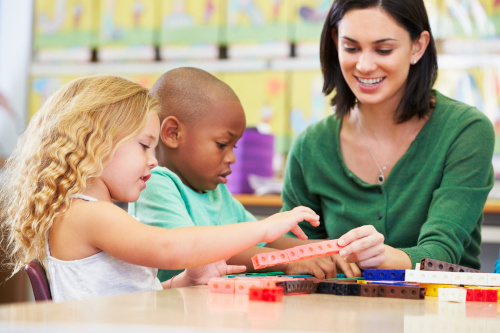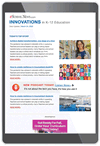# 3 ways to teach multi-sensory math

### Multi-sensory learning enables students to interact with the mathematics for deep, grounded learning

Learning mathematics is much more than memorization. Rote drill and practice have not shown to lead to significant improvements in mathematics abilities, but rather, using strategies that engage and strengthen the connections to different areas of the brain assists students in learning mathematics.

According to findings published in Teaching Children Mathematics, most students actually use a strategy to recall a fact. They are considered fluent if they can recall a fact within three seconds, which is a long time to be able to employ a strategy. For example, looking at the problem 19 + 6, students might move one from the 6 to make an easier, equivalent statement of 20 + 5.

Using multi-sensory learning to make sense of mathematics, as well as introducing students to strategies and tools such as the ones below, helps them become flexible thinkers and allows them to be fluid with numbers.

Counters and ten frames

Counters and ten frames help students “see” ten. Students use the counters and fill spaces in a ten frame. When they have all ten spaces full, they can see that they have ten. It is very satisfying!

If there are two empty spaces, they can see they have less than ten. Teachers can ask a question like, “How many more do you need to make ten?” Students can count on and realize they need two. Later, teachers can connect this visual model to the much more abstract number model of 8 + 2 = 10, and even 10 = 8 + 2.

Ten frames can be on photo-copied paper or drawn on personal whiteboards. There are also several versions available as digital manipulatives, such as this one, which allow students to drag digital counters into spaces. All function the same, allowing students to build ten, teen numbers, and multiples of ten, as well as ask questions and create equations.

Number lines

Number lines build on understanding quantity with counters, and connect that understanding to space. They are an important tool in all mathematics from K-16 and beyond.

Students use number lines to understand whole numbers and fractions, do computation, measure, graph, and find proportional relationships. However, students need to understand how a number on the number line represents the quantity of the distance traveled from zero. To land on the number two, students have to travel the entire spaces from 0 to 1, and then from 1 to 2.

Using counters on number paths helps students see the whole space needs to be covered. Transitioning to number lines, students can connect the quantities they used to cover the spaces with the movement from zero to the tick mark on the number line. This movement from zero provides the foundation for measurement with rulers.

Digital number lines, such as this one, allow students to drag arrows, or make jumps to land on the tick marks marking the number. This reinforces the connection of quantity with spatial reasoning and measurement. The measurement app on a mobile phone is also a great way for students to practice the spatial reasoning of quantity on the number line.

Classroom discussion

The most powerful multi-sensory learning tool in the mathematics classroom is talking. As students are working with quantities, teachers should ask them questions and listen to their answers. When working with the digital counters and ten frames to answer 8 + 5, teachers should ask them to explain their strategy or maybe follow up with a question like “what would 18 +5 look like?,” “Could we use a number line to solve that problem? Where would you land if you add 5?,” or “Show me on your computer!”

After giving students rulers to measure objects, teachers can also ask “How far did you travel from zero?” Sometimes zero isn’t located at the very end of the ruler, so this helps students see where to begin their measurement.

These three strategies are powerful because they help students process quantities and computation with different senses. Students are able to interact with physical or digital objects using touch and movement, connect numbers with distance and space on the number lines, and talk through – and listen to – the math, to process what they are learning. Multi-sensory learning enables students to interact with the mathematics for deep, grounded learning, which is so much more meaningful than merely memorizing steps.##Sign up for our K-12 newsletter